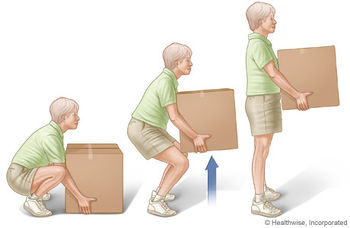# Energy vs power

In other languages: FrançaisFigure 1: Lifting a box requires a specific amount of energy, no matter how quickly the box is picked up. Lifting faster will change the amount of power but not the amount of energy.

Energy and power are closely related but are not the same physical quantity. Energy is the ability to cause change; power is the rate energy is moved, or used.

## Energy

main article

Energy is the ability to create a change, for example, creating motion. Tasks (like lifting a box) require an amount of energy to complete. A battery will hold a particular amount of energy; so will a given amount of fuel, like food.

The base unit of energy is the joule. This means that a task, like lifting a box in figure 1, takes a certain number of joules regardless of how quickly the box is picked up.

## Power

main article

Power is how fast energy is used or transmitted - power is the amount of energy divided by the time it took to use the energy. Its unit is the watt, which is one joule per second of energy used. A circular saw will draw a certain amount of power to run, and how quickly power is drawn from a battery determines how long its stored energy will last.

• P is the average power output, measured in watts (W)
• ΔEsys is the net change in energy of the system in joules (J) - also known as work.
• Δt is the duration - how long the energy use takes - measured in seconds (s)

Multiplying a value of power and the period of time over which it is used gives an amount of energy. This is why a kilowatt is a unit of power but a kilowatt-hour (1 kilowatt times 1 hour) is a unit of energy.

Tasks (like lifting a box) take a certain amount of energy (a certain number of joules), but the faster the task is done (the smaller Δt), the more power it takes (more watts).

### Engines vs. gas tanks

A car's engine determines how much power it can exert (this is a mechanical power often rated in horsepower, as opposed to the thermal power which is how fast it burns gasoline), while the amount of gasoline in the gas tank determines how much energy the engine has at its disposal. The greater the amount of gasoline, the longer the engine can run without running out of energy. The more power the engine is using (like when driving fast or revving the engine to accelerate quickly), the shorter the amount of time the chemical energy available in the gasoline will last. Here's a page on tricks for improving fuel economy.

### Capacitors vs. batteries

Capacitors often have a little bit of energy that can be discharged quite quickly. This short time means that they can have a fairly high power, even with a low amount of stored energy (this is useful with camera flashes, for example). Batteries, on the other hand, contain much more energy than a capacitor but discharge that energy far more slowly, meaning that they have a lower power output.

### An analogy with containers of waterFigure 2. The mug labelled 'Power' has a greater rate. The mug labelled 'Energy' has more water. This image represents how energy and power are different. Both have water and a flow rate.

Figure 2 shows a mug with a small amount of water being poured quickly and a jug holding a greater amount of water being poured more slowly. The mug is delivering more water in a given amount of time, but the total amount of water delivered will be less than the jug. The analogy is that the mug has higher power, but lower energy. The jug releases smaller amounts of liquid for a longer period of time. The analogy continues with the jug having a smaller power output but more energy. The mug releases all its water (energy) very quickly. Conversely, the jug holds much more water (energy), even if it is not losing it quickly.

## Authors and Editors

Ethan Boechler, Allison Campbell, James Jenden, Jason Donev
Last updated: September 27, 2021
Get Citation